Courses

# Test: Fourier Part - 3

## 10 Questions MCQ Test Signal and System | Test: Fourier Part - 3

Description
This mock test of Test: Fourier Part - 3 for Electrical Engineering (EE) helps you for every Electrical Engineering (EE) entrance exam. This contains 10 Multiple Choice Questions for Electrical Engineering (EE) Test: Fourier Part - 3 (mcq) to study with solutions a complete question bank. The solved questions answers in this Test: Fourier Part - 3 quiz give you a good mix of easy questions and tough questions. Electrical Engineering (EE) students definitely take this Test: Fourier Part - 3 exercise for a better result in the exam. You can find other Test: Fourier Part - 3 extra questions, long questions & short questions for Electrical Engineering (EE) on EduRev as well by searching above.
QUESTION: 1

### Consider a periodic signal x[n] with period N and FS coefficients X [k]. Determine the FS coefficients Y [k] of the signal y[n] given in question. Q. y[n] = (-1)n x[n], (assume that N is even)

Solution:

With N even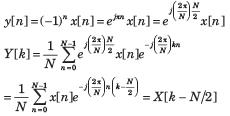QUESTION: 2

### Consider a discrete-time periodic signal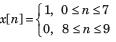with period N = 10. Also y[n] = x[n] - x[n - 1] Q. The fundamental period of y[n] is

Solution:

y[n] is shown is fig. S5.8.21. It has fundamental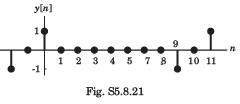period of 10.

QUESTION: 3

### Consider a discrete-time periodic signal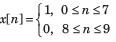with period N = 10. Also y[n] = x[n] - x[n - 1] Q. The FS coefficients of y[n] are

Solution: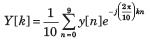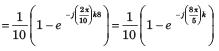QUESTION: 4

Consider a discrete-time periodic signalwith period N = 10. Also y[n] = x[n] - x[n - 1]
Q. The FS coefficients of x[n] are

Solution:

y [n] = x [n] - x [n - 1]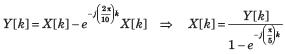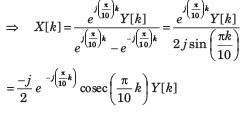QUESTION: 5

Consider a discrete-time signal with Fourier representation.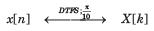In question the FS coefficient Y [k] is given. Determine the corresponding signal y[n] and choose correct option.
Q. Y [k] = X[k - 5] + X[k + 5]

Solution: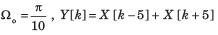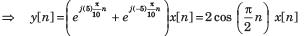QUESTION: 6

Consider a discrete-time signal with Fourier representation.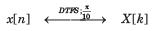In question the FS coefficient Y [k] is given. Determine the corresponding signal y[n] and choose correct option.
Q. Y [k] = cos (πk/5)X[k]

Solution: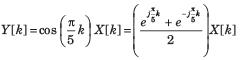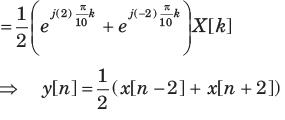QUESTION: 7

Consider a discrete-time signal with Fourier representation.In question the FS coefficient Y [k] is given. Determine the corresponding signal y[n] and choose correct option.
Q. Y [ k] = X [k] * X [k]

Solution:

Y [ k] = X [k] * X [k] ⇒ y[n] = x[n] x[n] = ( x[n])2

QUESTION: 8

Consider a discrete-time signal with Fourier representation.In question the FS coefficient Y [k] is given. Determine the corresponding signal y[n] and choose correct option.
Q. Y [k] = Re{ X [ k]}

Solution:

Y [k] = Re{ X[k]} ⇒ y[n] = Ev{ x[n]} =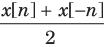QUESTION: 9

Consider a sequence x[n] with following facts :
1. x[n] is periodic with N = 6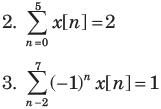4. x[n] has the minimum power per period among the set of signals satisfying the preceding three condition.
The sequence would be.

Solution: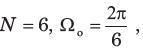From fact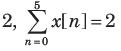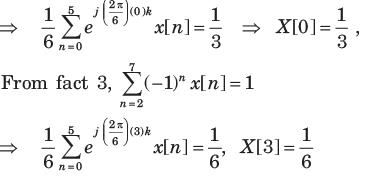By Parseval’s relation, the average power in x[n] is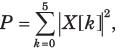The value of P is minimized by choosing
X  = X  = X [ 4 ] = X  = 0
Therefore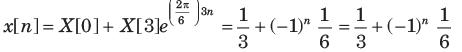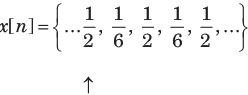QUESTION: 10

A real and odd periodic signal x[n] has fundamental period N = 7 and FS coefficients X [k]. Given that X  = j, X  = 2j, X  = 3j. The values of X ,X [ -1], X [-2 ], and X [-3] will be

Solution:

Since the FS coefficient repeat every N . Thus
X  = X , X [2 ] = X , X [ 3] = X 
The signal real and odd, the FS coefficient X [k] will be purely imaginary and odd. Therefore X  = 0
X [ -1] = -X , X [ -2 ] = - X [2 ], X [-3] = -X [ 3]
Therefore (D) is correct option.